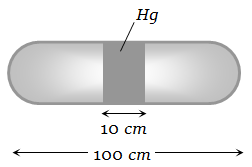The pressure and volume of saturated water vapour are P and V respectively. It is compressed isothermally thereby volume becomes V/2, the final pressure will be

1.  More than 2P

2.  P

3.  2P

4.  4P

High Yielding Test Series + Question Bank - NEET 2020

Difficulty Level:

If the intermolecular forces vanish away, the volume occupied by the molecules contained in 4.5 kg water at standard temperature and pressure will be

1.

2.

3.

4.

High Yielding Test Series + Question Bank - NEET 2020

Difficulty Level:

When an air bubble of radius ‘r’ rises from the bottom to the surface of a lake, its radius becomes 5r/4 (the pressure of the atmosphere is equal to the 10 m height of water column). If the temperature is constant and the surface tension is neglected, the depth of the lake is

1.  3.53 m

2.  6.53 m

3.  9.53 m

4.  12.53 m

High Yielding Test Series + Question Bank - NEET 2020

Difficulty Level:

A horizontal uniform glass tube of 100 cm, length sealed at both ends contain 10 cm mercury column in the middle. The temperature and pressure of air on either side of mercury column are respectively 81°C and 76 cm of mercury. If the air column at one end is kept at 0°C and the other end at 273°C, the pressure of air which is at 0°C is (in cm of Hg)1.  76

2.  68.2

3.  102.4

4.  122

High Yielding Test Series + Question Bank - NEET 2020

Difficulty Level:

At standard temperature and pressure the density of a gas is  and the speed of the sound in gas is 330 m/sec. Then the degree of freedom of the gas will be

1.  3

2.  4

3.  5

4.  6

High Yielding Test Series + Question Bank - NEET 2020

Difficulty Level:

The temperature of 5 moles of a gas which was held at constant volume was changed from $100°\mathrm{C}$ to $120°\mathrm{C}$. The change in internal energy was found to be 80 Joules. The total heat capacity of the gas at constant volume will be equal to

1.  8 J/K

2.  0.8 J/K

3.  4.0 J/K

4.  0.4 J/K

High Yielding Test Series + Question Bank - NEET 2020

Difficulty Level:

The temperature at which the r.m.s. speed of hydrogen molecules is equal to escape velocity on earth surface, will be

1.  1060 K

2.  5030 K

3.  8270 K

4.  10063 K

High Yielding Test Series + Question Bank - NEET 2020

Difficulty Level:

Inside a cylinder having insulating walls and closed at ends is a movable piston, which divides the cylinder into two compartments. On one side of the piston is a mass m of a gas and on the other side a mass 2 m of the same gas. What fraction of volume of the cylinder will be occupied by the larger mass of the gas when the piston is in equilibrium ? Consider that the movable piston is conducting so that the temperature is the same throughout

1.  $\frac{1}{4}$

2.  $\frac{1}{3}$

3.  $\frac{1}{2}$

4.  $\frac{2}{3}$

High Yielding Test Series + Question Bank - NEET 2020

Difficulty Level:

The diameter of oxygen molecules is  The Vander Waal's gas constant 'b' in ${\mathrm{m}}^{3}/\mathrm{mol}$ will be

1.  $3.2$

2.  $16$

3.  $32×{10}^{-4}$

4.  $32×{10}^{-6}$

High Yielding Test Series + Question Bank - NEET 2020

Difficulty Level:

The temperature of the mixture of one mole of helium and one mole of hydrogen is increased from $0°\mathrm{C}$ to $100°\mathrm{C}$ at constant pressure. The amount of heat delivered will be

1.  600 cal

2.  1200 cal

3.  1800 cal

4.  3600 cal